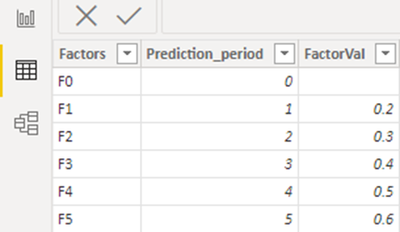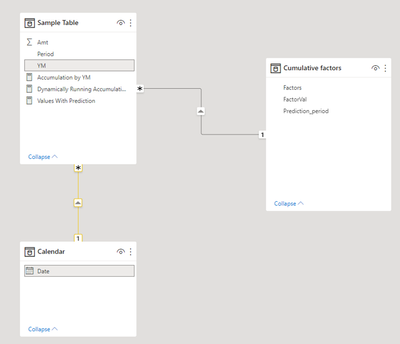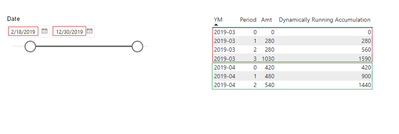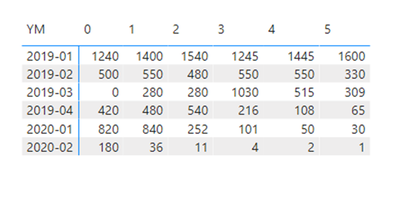cancel
Showing results for
Did you mean:## How to Calculate Cumulative Values with DAX

Scenario:
In some scenarios, you may need to do some accumulated calculations such as cumulative sum which is also called running total or multiplication with DAX for subsequent visualizations. And in this article, I will show you several scenarios regarding accumulation and tell you how to achieve it.

Table used:
Sample Table:Expected Result:
Cumulative sum result:Cumulative multiplication result:Calculate cumulative sum:
In the past, we did receive many requirements of getting the cumulative sum/running total of some values and hoping the calculation is carried by a specific group. With the following DAX code, it’s easy to implement it.

Detailed Steps:
1. Remove all context filters in the table ‘Sample Table’ except filters that have been applied to the ‘Sample Table’[YM] column for we hope the accumulation is evaluated and the result is only affected by each Year Month.

``ALLEXCEPT ( 'Sample Table', 'Sample Table'[YM] )``

2. Use Filter function to manipulate the context to get filtered rows for calculation. In this situation, the Boolean expression we need is 'Sample Table'[Period] <= MAX ( 'Sample Table'[Period] ), which will return a subset of the ‘Sample Table’ that only contains current row and previous row in the same group of YM.

``````FILTER (
ALLEXCEPT ( 'Sample Table', 'Sample Table'[YM] ),
'Sample Table'[Period] <= MAX ( 'Sample Table'[Period] )
)``````

3. Sum ‘Sample Table’[Amt] based on the steps above and the complete DAX code is as follows.

``````Accumulation by YM =
CALCULATE (
SUM ( 'Sample Table'[Amt] ),
FILTER (
ALLEXCEPT ( 'Sample Table', 'Sample Table'[YM] ),
'Sample Table'[Period] <= MAX ( 'Sample Table'[Period] )
)
)``````

Result:In addition to the basic accumulation, chances are that you need dynamically running total and normally dynamically change with dates. Then we need an extra Date table that can be generated with the Calculated Table below.

``Calendar = CALENDAR(MIN('Sample Table'[YM]),MAX('Sample Table'[YM]))``

Build a relationship between ‘Sample Table’ table and ‘Calendar’ table using YM column from ‘Sample Table’ table and Date column from the other side.With another measure, you can see the running total becomes dynamic as expected.

``````Dynamically Running Accumulation =
CALCULATE (
[Accumulation by YM],
FILTER (
'Sample Table',
'Sample Table'[YM] >= MIN ( 'Calendar'[Date] )
&& 'Sample Table'[YM] <= MAX ( 'Calendar'[Date] )
)
)``````

Result:Calculate cumulative multiplication :
After the introduction of some cumulative sum scenarios, let’s talk about calculating cumulative multiplication. In this part, we need to make predication for the blank cells and the final predicted values results from the latest value and a series of cumulative factors. To complete this type of multiplication with DAX, there are two DAX Functions that are worth knowing before the calculation.
PRODUCT: Returns the product of the numbers in a column.
PRODUCTX: Returns the product of an expression evaluated for each row in a table.

Detailed Steps:
1. Get the min periods from which the values are blank for each Year Month group with the ‘Min_No_Val_Period’ variable

``````VAR Min_No_Val_Period =
CALCULATE (
MAX ( 'Sample Table'[Period] ),
ALLEXCEPT ( 'Sample Table', 'Sample Table'[YM] )
) + 1
``````2. Get the base multiplier for every Year Month group using the ‘FactVal’ variable

``````VAR FactVal =
CALCULATE (
MAX ( 'Sample Table'[Amt] ),
FILTER (
ALL ( 'Cumulative factors' ),
'Cumulative factors'[Prediction_period]
< MAX ( 'Cumulative factors'[Prediction_period] )
)
)
``````3. Fetch factor values from ‘Cumulative factors’ table, and calculate predicted values for empty cells with PRODUCTX function. As for non-empty cells, fill them with actual Amt values. The complete dax code looks like this.

``````Values With Prediction =
VAR Amt =
SUM ( 'Sample Table'[Amt] )
VAR MaxPeriod =
CALCULATE (
MAX ( 'Sample Table'[Period] ),
ALLEXCEPT ( 'Sample Table', 'Sample Table'[YM] )
) + 1
VAR FactVal =
CALCULATE (
MAX ( 'Sample Table'[Amt] ),
FILTER (
ALL ( 'Cumulative factors' ),
'Cumulative factors'[Prediction_period]
< MAX ( 'Cumulative factors'[Prediction_period] )
)
)
VAR factors =
MAX ( 'Cumulative factors'[FactorVal] )
RETURN
IF (
ISBLANK ( Amt ),
PRODUCTX (
FILTER (
ALL ( 'Cumulative factors' ),
'Cumulative factors'[Prediction_period] >= MaxPeriod
&& 'Cumulative factors'[Prediction_period]
<= MAX ( 'Cumulative factors'[Prediction_period] )
),
'Cumulative factors'[FactorVal]
) * FactVal,
Amt
)``````

Result:So far, we have discussed several cumulative scenarios and provided solutions to implement them with dax. Hope this article will be helpful when you need to do some similar calculations.

Author: Caiyun Zheng

Reviewer: Kerry Wang & Ula Huang

Top Kudoed Posts
Latest Articles